Function Repository Resource:

# LocalMinima

Compute the local minima of an expression with respect to the given variables

Contributed by: Wolfram|Alpha Math Team
 ResourceFunction["LocalMinima"][expr,x] computes the local minima of expr with respect to x. ResourceFunction["LocalMinima"][expr,{x,y,…}] computes the local minima of expr with respect to multiple variables. ResourceFunction["LocalMinima"][{expr,const},{x,y,…}] computes the local minima of expr subject to the constraint const.

## Details and Options

ResourceFunction["LocalMinima"] returns a list with each minimum in the form {fmin,{xxmin,yymin,}}.
The const can contain equations, inequalities or logical combinations of these.
ResourceFunction["LocalMinima"] only returns results when there is a bounded minimum.

## Examples

### Basic Examples (2)

Compute the local minima of a polynomial:

 In:=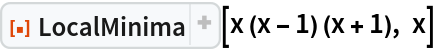Out=Plot them:

 In:=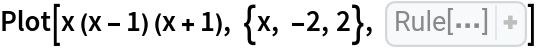Out=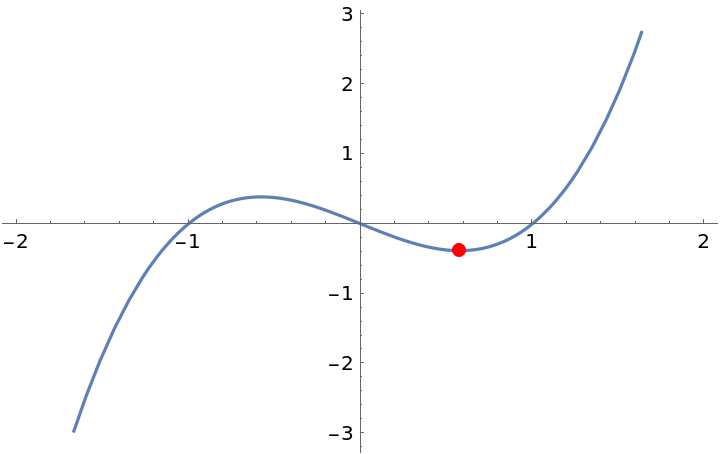### Scope (2)

Use a constraint in order to reduce the domain upon which minima can be found:

 In:=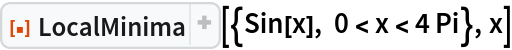Out=Compute the minima of a function of two variables:

 In:=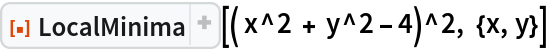Out=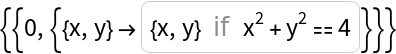### Possible Issues (1)

LocalMinima may return duplicate results for periodic functions:

 In:=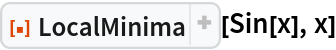Out=## Publisher

Wolfram|Alpha Math Team

## Version History

• 2.0.0 – 23 March 2023
• 1.0.0 – 18 September 2020

## Author Notes

To view the full source code for LocalMinima, run the following code:

 In:=